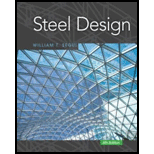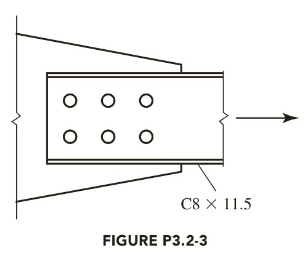# A C 8 × 11.5 is connected to a gusset plate with 7 8 -inch-diameter bolts as shown in Figure P3.2-3. The steel is A572 Grade 50. If the member is subjected to dead load and live load only, what is the total service load capacity if the live-to-dead load ratio is 3? Assume that A e = 0.85 A n . a . Use LRFD. b . Use ASD.### Steel Design (Activate Learning wi...

6th Edition
Segui + 1 other
Publisher: Cengage Learning
ISBN: 9781337094740

#### Solutions

Chapter
Section### Steel Design (Activate Learning wi...

6th Edition
Segui + 1 other
Publisher: Cengage Learning
ISBN: 9781337094740
Chapter 3, Problem 3.2.3P
Textbook Problem
41 views

## A C 8 × 11.5 is connected to a gusset plate with 7 8 -inch-diameter bolts as shown in Figure P3.2-3. The steel is A572 Grade 50. If the member is subjected to dead load and live load only, what is the total service load capacity if the live-to-dead load ratio is 3? Assume that A e = 0.85 A n .a. Use LRFD.b. Use ASD.To determine

(a)

### Explanation of Solution

Given:

C8×11.5 gusset plates with 78 bolts.

LL=3DL.

Concept Used:

Write the expression for the factored strength in yielding.

P=Φy×Pny ...... (I)

Write the expression for the factored strength in rupture.

P=Φf×Pnf ...... (II)

Here the strength of the material is P, the resistance factor for yielding is Φy, the resistance factor for rupture is Φf, the nominal strength in yielding is Pny, and the nominal strength in rupture is Pnr

The design strength of LRFD is the minimum of Equation (I) and (II).

Write the expression for the nominal strength in yielding.

Pny=Fy×Ag ...... (III)

Here, the yield strength in yielding is Fy, the gross area of the member is Ag.

Write the expression for the gross area of the member.

Ag=L×t ...... (IV)

Here, the length of the member is L, the thickness of the member is t.

Write the expression for the nominal strength in rupture.

Pnf=Fu×Ae ...... (V)

Here, the yield strength in rupture is Fu, the effective area is Ae.

Given that, Ae=An, hence,

Ae=An=AgAholes ...... (VI)

Here, the area of the holes is Aholes, the net area is An.

Write the expression for the area of the holes.

Aholes=t×dholes ...... (VII)

Here the diameter of the holes is dholes.

Write the expression for the diameter of the holes.

dholes=d+18 ...... (VIII)

Here the diameter of the bolts is d and 18 is the allowance for side clearance for bolts less than 1.

For the A36 steel resistance factors and the yield strength values are,

Φy=0.90Φf=0.75Fy=50ksiFu=65ksi

Calculation:

Calculate the diameter of holes.

Substitute 78 for d in Equation (VIII).

dholes=78+18=1in

Calculate the gross area.

As per the codes for the section C8×11.5, the gross area is Ag=3.37in2.

Calculate the area of holes.

Substitute 0.22in for t and 1in for dholes in Equation (VII)

To determine

(b)

The service load using Allowable Strength Design (ASD).

### Still sussing out bartleby?

Check out a sample textbook solution.

See a sample solution

#### The Solution to Your Study Problems

Bartleby provides explanations to thousands of textbook problems written by our experts, many with advanced degrees!

Get Started

Find more solutions based on key concepts
What are the basic characteristics of nonlinear models?

Engineering Fundamentals: An Introduction to Engineering (MindTap Course List)

How is a blade server different from a regular server?

Principles of Information Systems (MindTap Course List)

Convert 10,000 ft-lbf of energy into BTU, Joules, and kilojoules.

Fundamentals of Chemical Engineering Thermodynamics (MindTap Course List)

Briefly discuss the OLAP architectural styles with and without data marts.

Database Systems: Design, Implementation, & Management

List the sections of a system design specification, and describe the contents.

Systems Analysis and Design (Shelly Cashman Series) (MindTap Course List)

Describe how to use a search engine. What are some ways you can refine a search?

Enhanced Discovering Computers 2017 (Shelly Cashman Series) (MindTap Course List)

If your motherboard supports ECC DDR3 memory, can you substitute non-ECC DDR3 memory?

A+ Guide to Hardware (Standalone Book) (MindTap Course List)

What do the two pressure gauges on a regulator show?

Welding: Principles and Applications (MindTap Course List)